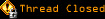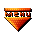# Topic awaiting preservation: My brain is melting! (AKA Calculating form value avg) (Page 1 of 1)Dufty
Paranoid (IV) Inmate

From: Where I'm from isn't where I'm at!
Insane since: Jun 2002posted 10-24-2002 12:29

Hi again.

I have a small problem, and was hoping someone could point out where I'm going wrong.
(It's worth mentioning that Math isn't a strongpoint, and that may be the root of my problem!)

Below is a block of code for calculating the average score for a block of inputs, but I need it to reduce the number averaged by, if there is an N/A in any of the selection fields.

For some reason, I get minus numbers, so I'm obviously doing something wrong... just can't for the life of me see what.
(Is it even valid code to have if statements inside if statements???)

In advance, many many thanks for taking the time to look at this for me.
-Dufty

code:
```<html>
<script LANGUAGE = JavaScript>

function calculateTotals(x) {
with (document.forms["myForm"])
{

var totalScore = 0;
var divIde = 0;

if (x == 1) {

divIde = 3

if (element1.value == "0" ) {
divIde = -1
}

if (element2.value.value == "0") {
divIde = -1
}

if (element3.value == "0") {
divIde = -1
}

totalScore = Number(element1.value) + Number(element2.value) + 	Number(element3.value);

blockAvg1.value = avgCalc(totalScore/divIde,2);
}

function avgCalc(num,pow) {

num *=Math.pow(10,pow);
num = (Math.round(num)/Math.pow(10,pow)) + "";

return num;

}

}
}
</script>
<body>
<form name=myForm>
<table>

<tr valign="top">
<td> Calculate Average</td>
<td> <input type="button" value="Block Average" onClick=calculateTotals(1)>
<input name="blockAvg1" type="text" value="?"> </td>
</tr>

<tr valign="top">
<td>
<div align="left">Element 1</div></td>
<td> <div align="left">
<select name="element1">
<option value="0" selected>N/A</option>
<option value="1">1</option>
<option value="2">2</option>
<option value="3">3</option>
<option value="4">4</option>
<option value="5">5</option>
</select>
</div>
</td>
</tr>

<tr valign="top">
<td> <div align="left">Element 2</div></td>
<td> <div align="left">
<select name="element2">
<option value="0" selected>N/A</option>
<option value="1">1</option>
<option value="2">2</option>
<option value="3">3</option>
<option value="4">4</option>
<option value="5">5</option>
</select>
</div>
</td>
</tr>

<tr valign="top">
<td><div align="left">Element 3</div></td>
<td> <div align="left">
<select name="element3">
<option value="0" selected>N/A</option>
<option value="1">1</option>
<option value="2">2</option>
<option value="3">3</option>
<option value="4">4</option>
<option value="5">5</option>
</select>
</div>
</td>
</tr>
</table>
</form>
</body>
</html>```

WOW! Just posting it here revealed one problem:

code:
```if (element1.value == "0" ) {
divIde = -1
}

if (element1.value == "0" ) {
divIde = divIde -1
}```

But still inaccurate....

[This message has been edited by Dufty (edited 10-24-2002).]

Dufty
Paranoid (IV) Inmate

From: Where I'm from isn't where I'm at!
Insane since: Jun 2002posted 10-24-2002 13:48

I realy think I'm going mad!

Need to see if this code differs from the above, if not, then why did it suddenly work???

code:
```var totalScore = 0;
var divIde = 0;

if (x == 1) {

divIde = 3;

if (element1.value == "0" ) {
divIde = divIde -1;
}

if ([b]element2.value[/b] == "0") {
divIde = divIde -1;
}

if (element3.value == "0") {
divIde = divIde -1;
}

totalScore = Number(element1.value) + Number(element2.value) + 	Number(element3.value);

blockAvg1.value = avgCalc(totalScore/divIde,2);
}```

Aaaaaargh - it hurts!!! - But I spotted the error though! *Phew*

[This message has been edited by Dufty (edited 10-24-2002).]

Dufty
Paranoid (IV) Inmate

From: Where I'm from isn't where I'm at!
Insane since: Jun 2002posted 10-24-2002 14:37

Oh, how the plot thickens!!!

Got the previous script to work fine, but has caused a new problem:
Assuming there is no 'score' to average out (i.e. element1.value == "0" + element2.value == "0" + element3.value == "0"), then blockAvg1 shows NaN

I'm now struggling to calculate an average for all blockAvg(x) fields on the form, in order to show a TOTAL score.
(Using the same Math calculator to work out the average)

code:
```if (blockAvg1.value == NaN) {
divIde = divIde -1;
}```

Is this the right way of finding the NaN value?
(I've also tried null - didn't work either)

Currently, if even one of the blockAvg fields == NaN - the TOTAL score for the page == NaN !

Bugger!

Thanks again... Dufty

[This message has been edited by Dufty (edited 10-24-2002).]

Veneficuz
Paranoid (IV) Inmate

From: A graveyard of dreams
Insane since: Mar 2001posted 10-24-2002 16:34

To get rid of the NaN values all together you could do this:

code:
```if (totalScore == 0) blockAvg1.value = 0;
else blockAvg1.value = avgCalc(totalScore/divIde,2);```

If you still want the NaN value to show, but don't want to calculate with it you can sort out the NaN like this:

code:
```if (blockAvg1.value == "NaN") {
divIde = divIde -1;
}```

Was that what you were looking for?

_________________________
Anyone who has lost track of time when using a computer knows the propensity to dream, the urge to make dreams come true and the tendency to miss lunch.
- copied from the wall of cell 408 -

Dufty
Paranoid (IV) Inmate

From: Where I'm from isn't where I'm at!
Insane since: Jun 2002posted 10-24-2002 16:59

I'd already tried the second idea, to no avail, but the code for skipping it altogether and inserting "0" works a treat.

A thousand thanks bestowed upon thee!Slimeposted 10-24-2002 19:07